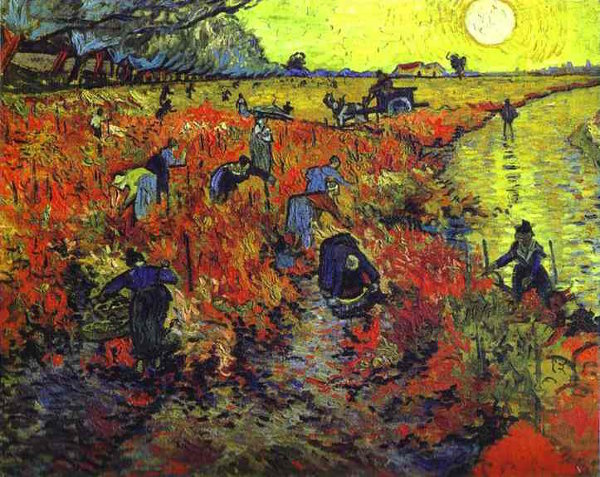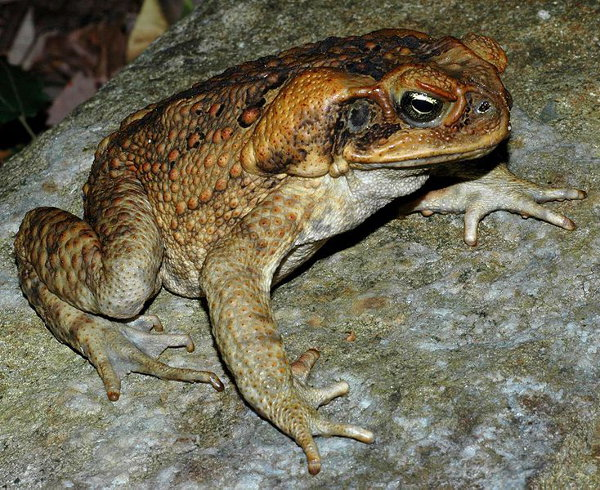# Introductory Percentages

Percentages are used in:

• bank savings, loans and credit cards
• discounts and profits of store transactions
• population increase and decrease

It is helpful to remember that:

• "Per" means "divide" and "Cent" means "100"
• To change a fraction to a decimal, divide using a calculator.
• To change a decimal to a percent, multiply by 100.

 LEARN THESE OFF BY HEART FRACTION DECIMAL PERCENTAGE 1⁄2 0.5 50% 1⁄4 0.25 25% 3⁄4 0.75 75% 1⁄10 0.1 10% 1⁄5 0.2 20% 3⁄10 0.3 30% 2⁄5 0.4 40% 3⁄5 0.6 60% 7⁄10 0.7 70% 4⁄5 0.8 80% 9⁄10 0.9 90% 1 1 100%

## Example One - Changing a Fraction to a Percentage

Change 38 to a percentage.

38 = 0.375 (Use a calculator to divide.)
38 × 100 = 37.5%
0.375 × 100 = 37.5%

## Example Two - Changing a Percentage to a Decimal

Change 245% to a decimal.

245% = 2.45 (Divide by 100 or move the decimal point 2 places to the left.)

## Example Three - Changing a Percentage to a Proper Fraction

Change 96% to a fraction.

96% = 96100 = 2425 (Simplify the fraction by dividing the numerator and the denominator by 4.)

## Example Four - Changing a Percentage to a Mixed Number

Change 235% to a fraction.

235%
= 235100
= 4720 (Simplify the fraction by dividing the numerator and the denominator by 5.)
= 2 720

## Questions

Q1. Change 78 to a decimal and a percentage.
Q2. Change 24% to a decimal.
Q3. Change 48% to a fraction.

A1. 0.875
A2. 0.24
A3. 1225

## Example Five - Finding Percent Change

Divide the amount of change by the original amount.
The International Whaling Commission estimates that Humpback Whale numbers have increased from 50 000 to 55000 in 2009-2011. What percent increase is this?

Amount of increase = 55000 – 50000 = 5000 whales

 Percent increase = Amount of increase × 100 Original Amount
 = 5000 × 100 50000

= 10% increase

## Did You Know That...?This painting entitled "Red Vineyards" by Dutch painter, Vincent van Gogh, was the only painting he sold in his lifetime. It sold in 1890 for about \$1500 and is now worth millions.

## Example Six - Finding the Original Amount

A discount of 20% for a soccer jersey was given at a sports store. This discount is \$13.00. What was the original price before the discount?
(Hint: Turn the fraction upside down and multiply.)

The original price is always 100%.

 Original price = 100 × 43 20

= \$65

## QuestionsQ1. The introduction of the Cane Toad to Australia was a curse. It is poisonous, kills native wildlife, is a prolific breeder and has no predators. From 1990 to 2010, the estimated area it inhabits has increased from 120 000 to 150 000 square kilometres. What percent increase is this?

Q2. The habitat of the African Mountain Gorilla, now an endangered animal, is being destroyed to mine Coltan, the Rare Earth Metal ore needed for mobile 'phone technology. The population was estimated at 480 gorillas in 2010. This is a 25% decrease since 2003. What is the original population in 2003?